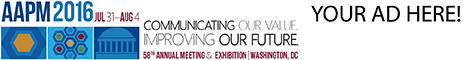# Program Information

## Rapid Direct Aperture Optimization Via Piecewise Linear Dose Calculation Based On the Dose Influence MatrixX Zeng

## Presentations

#### TH-EF-BRD-6 (Thursday, July 16, 2015) 1:00 PM - 2:50 PM Room: Ballroom D

Purpose: Direct aperture optimization (DAO) considers the direct optimization of aperture leaf positions and weights, instead of a two-step procedure for intensity modulated radiation therapy (IMRT), i.e. first fluence map optimization and then leaf sequencing algorithm. However, the use of the Monte Carlo (MC) method in DAO is often time-consuming. This work aims to develop a rapid DAO algorithm.

Methods: The proposed method is based on the dose influence matrix. That is the leaf position variable is a piecewise linear function of the dose influence matrix. Specifically, the piecewise linear dose calculation model with respect to left and right leaf positions of a aperture consists of three terms: (i) the total dose contribution from the bixels that are fully between the left and right leaf positions; (ii) the partial dose contribution from the leftmost bixel that the left leaf falls into; (iii) the partial dose contribution from the rightmost bixel that the right leaf falls into. As a result, this model is piecewise linear with respect to the leaf positions.
Then instead of MC, this piecewise linear dose calculation engine based on the dose influence matrix is incorporated into the simulated annealing algorithm for DAO. As a result of rapid dose calculation based on the dose influence matrix, the speed of DAO is improved significantly.

Results: The proposed algorithm was tested on the TG119 phantom from the MGH public dataset.

Conclusion: Rapid DAO algorithm is developed with the dose calculation engine that replaces MC by the piecewise linear dose calculation based on the dose influence matrix.

Funding Support, Disclosures, and Conflict of Interest: The authors were partially supported by the NSFC (#11405105), the 973 Program (#2015CB856000) and the Shanghai Pujiang Talent Program (#14PJ1404500).

Contact Email: